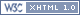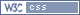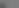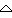Welcome, Guest. Please login or register. May 18, 2019, 11:49:52 PM 1 Hour 1 Day 1 Week 1 Month Forever Login with username, password and session length Forum changes: Editing of posts has been turned off until further notice.
Search:     Advanced search
 46709 Posts in 5588 Topics by 13299 Members Latest Member: - Jason DAngelo Most online today: 60 - most online ever: 429 (November 03, 2007, 04:35:43 AM)
 Pages: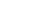Author Topic: Probability Puzzle. Attack vs Defense rolls, w/ modifier.  (Read 2642 times)
Maugh
Member

Posts: 24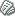« on: July 15, 2011, 11:32:36 AM »

So, I'm looking at dice probabilities, and I have a puzzle for you folks, which I could use some help with.  It has to do with balancing attack and defense probabilities.

Take a single one vs one attack mechanic, where an attacker rolls one die, which can be any of the standard dice, d4 to d12, and the defender, likewise, is rolling one die, d4 to d12.  Also take into account that the attacker can gain anywhere from a -4 to a +4 bonus on that attack.

What would be a formula to determine the probability of the attacker rolling even or higher than the defender, counting the modifier?

Sticky problem.  I could brute-force it in excel, but there has to be a formula out there that could mimic this instead of trying to string out every possibility and add the probabilities.Logged
horomancer
Member

Posts: 131« Reply #1 on: July 17, 2011, 03:15:24 AM »

I suck at probability too so i use http://anydice.com

It shouldn't be hard to figure out with fractions.
You have a total number of possibilities equal to one die type multiplied against the other. The probability will be equal to all the rolls that do what you want them to do expressed as fractions. So lets say you have an attacker and defender, each with d6 for there dice, they have a total of 36 possible combinations of dice. Since the attacker wins on 0 and anything greater than 0, he has a slight advantage and his number can be express as !6. This means he wins on the 6 possible rolls that equal 0, the 5 possible rolls that equal +1, 4 that equal +2, 3 =+3, 2=+4 and the one roll that equals +5. The defender would win on !5, meaning all the other possible rolls.

When you get multiple dice type in the mix you have to be careful not to get your attacker and defender values swapped.

Lets say the attack has a d6 and the defender has a d8. There are (6*8) or 48 possible outcomes.
The attackers % chance of success is equal to !6 but there will be time when his d6 just can't beat the d8, when it rolls a 7 or 8 and this is reflected by the larger denominator

!6=6+5+4+3+2+1= 21     21/48= 0.4317 or 43%

Now what if we had the attacker with the larger die? D8 vs d6. setting up !8 wouldn't yield us an accurate result and neither would subtracting 43% from 100%. the reason being that the dice rolls that yield 0 are are in the dead centre of the bell curve and are always on the side of the attack, rather than being neutral. To set the math up proper you subtract the difference in range from the larger !n value
in this case it would be !8 - !(8-6)=(8+7+6+5+4+3+2+1) - (2+1)= 33        33/48= 0.6875 or 68.75%

Now if you add those fractions together you see you get a total of 54/48. The reason this is effectively over 100% is that the rolls that equal 0 are effectively counted twice, since they are always in the attackers favor. 54-48=6   6/48= 0.125
So 12.5% of the time you roll a 0 when you have a d6 and a d8

Now for the integer.Logged
horomancer
Member

Posts: 131« Reply #2 on: July 17, 2011, 04:23:20 AM »

Why is there no edit button? anyways the integer

The integer that is the bonus of +4 to -4 won't change the shape of your bellcurve, but re-centre it. This is kinda tricky and i don't have good math to represent it but i can make up some rules based on observing the way the numbers change in anydice.

1. if the attacker's dice is low, it is at the bottom of the plateau
2. if the attackers dice is high it's at the top of the plateau
3. the plateau is equal to the difference in the die size.
4. The smallest !n value is used.

What this means is that for ever +1 bonus you add a value equal to !n of the smallest die to the attack value. For ever step of plateau you add the MAXIMUM !n of the smallest die until the plateau ends, then you step it down

So lets say you have a +3 to attack and are rolling a d6 vs a d6. Since the dice are equal there is no plateau, and the math would look like this-

((6+5+4+3+2+1)+(5+4+3))/(6*6) = (21+12)/36 = 33/36 = 0.91666 or 92%
{            }
These 3 digits are from the +3 attack bonus. They start at !6 and go down steadily. However since there was no plateau since the dice are equal they start at 5

Now, same attack bonus, but with the attacker having d4 and the defender having d10
The plateau is equal to 10 - 4 = 6, This means that the first bonus points up to the 6th bonus will give the attacker the maximum !n of the smallest dice

((!4)+(4+4+4))/(4*10) = (10+12)/40 = 22/40 = 0.55 = 55%
{          }
These 3 digits come from the +3 attack bonus, and are the maximum value of !n for the d4, There are three 4's since the plateau between these dice is 6. The attack bonus would have to be a +7 for the value to drop from 4 to 3. at +8 the bonus would drop to 2 and only be 1 at +9 attack bonus

OK now d10+3 vs d4
Plateau is 6 again, but the attacker is the high dice, meaning he is at the top of the plateau already so

((!10 - !6)+(3+2+1))/(4*10) =  (34+6)/40 = 40/40 = 1 or 100%

Here we see that the same bonus does little for a strong attacker, as without the bonus his odds would have been 90%
In the previous scenario the d4+3 more than doubled the attacker's chances of hitting, while in this scenario it only added 10%Logged
 Pages: 
Jump to: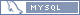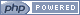Oxygen design by Bloc